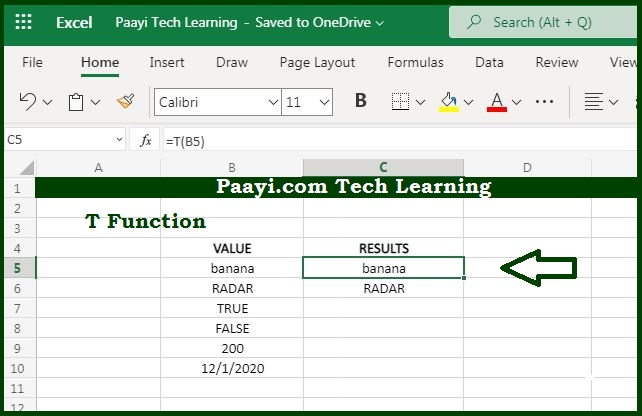# Learn How to Use Microsoft Excel T Function

Written by | 0 Comments | 739 Views

In this article, you will learn how to use the Microsoft Excel "T" function and its prime function in Microsoft Excel. You will also get to know the Microsoft Excel "T" function return value and syntax with the help of some examples.

Microsoft Excel T Function

The main use of the Microsoft Excel "T" Function is to filter the text value only. So, you can able to text when given a text value and an empty string (" ") for numbers, dates, and the logical values TRUE and FALSE. The T function is provided for compatibility with other spreadsheet programs and is generally not required.

Return Value of T Function

The return value will be the text when text.

Syntax of T Function

=T(value)

Where the arguments:

value: It is the value to return as a text.

## How to Use Microsoft Excel T Function?The main use of the Microsoft Excel "T" Function is to filter the text value only. So, you can able to text when given a text value and an empty string (" ") for numbers, dates, and the logical values TRUE and FALSE. The T function is provided for compatibility with other spreadsheet programs and is generally not required.

In most cases, using the T function is unnecessary, because Microsoft Excel automatically converts values when needed. The T function is provided for compatibility with other spreadsheet programs.